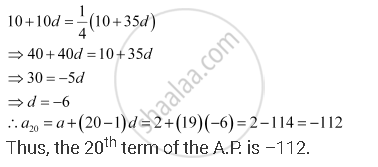CBSE (Arts) Class 11CBSE
Share

# In an A.P, the First Term is 2 and the Sum of the First Five Terms is One-fourth of the Next Five Terms. Show that 20th Term is –112. - CBSE (Arts) Class 11 - Mathematics

ConceptArithmetic Progression (A.P.)

#### Question

In an A.P, the first term is 2 and the sum of the first five terms is one-fourth of the next five terms. Show that 20th term is –112.

#### Solution

First term = 2

Let d be the common difference of the A.P.

Therefore, the A.P. is 2, 2 + d, 2 + 2d, 2 + 3d, …

Sum of first five terms = 10 + 10d

Sum of next five terms = 10 + 35d

According to the given condition,Is there an error in this question or solution?

#### APPEARS IN

NCERT Solution for Mathematics Textbook for Class 11 (2018 to Current)
Chapter 9: Sequences and Series
Q: 3 | Page no. 185

#### Video TutorialsVIEW ALL 

Solution In an A.P, the First Term is 2 and the Sum of the First Five Terms is One-fourth of the Next Five Terms. Show that 20th Term is –112. Concept: Arithmetic Progression (A.P.).
S